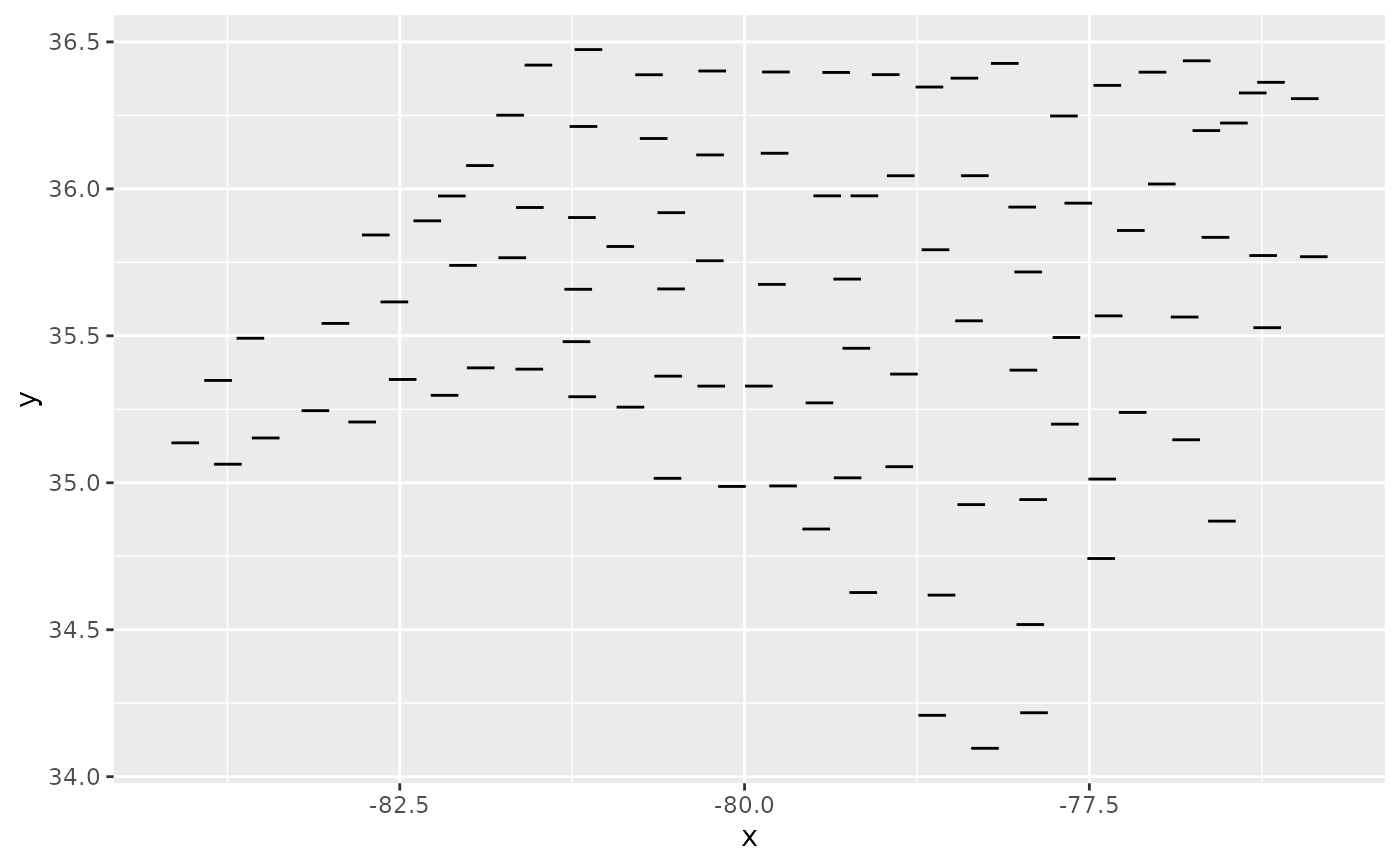stat_sf_coordinates() extracts the coordinates from 'sf' objects and summarises them to one pair of coordinates (x and y) per geometry. This is convenient when you draw an sf object as geoms like text and labels (so geom_sf_text() and geom_sf_label() relies on this).

## Usage

stat_sf_coordinates(
mapping = aes(),
data = NULL,
geom = "point",
position = "identity",
na.rm = FALSE,
show.legend = NA,
inherit.aes = TRUE,
fun.geometry = NULL,
...
)

## Arguments

mapping

Set of aesthetic mappings created by aes(). If specified and inherit.aes = TRUE (the default), it is combined with the default mapping at the top level of the plot. You must supply mapping if there is no plot mapping.

data

The data to be displayed in this layer. There are three options:

If NULL, the default, the data is inherited from the plot data as specified in the call to ggplot().

A data.frame, or other object, will override the plot data. All objects will be fortified to produce a data frame. See fortify() for which variables will be created.

A function will be called with a single argument, the plot data. The return value must be a data.frame, and will be used as the layer data. A function can be created from a formula (e.g. ~ head(.x, 10)).

geom

The geometric object to use to display the data, either as a ggproto Geom subclass or as a string naming the geom stripped of the geom_ prefix (e.g. "point" rather than "geom_point")

position

Position adjustment, either as a string naming the adjustment (e.g. "jitter" to use position_jitter), or the result of a call to a position adjustment function. Use the latter if you need to change the settings of the adjustment.

na.rm

If FALSE, the default, missing values are removed with a warning. If TRUE, missing values are silently removed.

show.legend

logical. Should this layer be included in the legends? NA, the default, includes if any aesthetics are mapped. FALSE never includes, and TRUE always includes. It can also be a named logical vector to finely select the aesthetics to display.

inherit.aes

If FALSE, overrides the default aesthetics, rather than combining with them. This is most useful for helper functions that define both data and aesthetics and shouldn't inherit behaviour from the default plot specification, e.g. borders().

fun.geometry

A function that takes a sfc object and returns a sfc_POINT with the same length as the input. If NULL, function(x) sf::st_point_on_surface(sf::st_zm(x)) will be used. Note that the function may warn about the incorrectness of the result if the data is not projected, but you can ignore this except when you really care about the exact locations.

...

Other arguments passed on to layer(). These are often aesthetics, used to set an aesthetic to a fixed value, like colour = "red" or size = 3. They may also be parameters to the paired geom/stat.

## Details

coordinates of an sf object can be retrieved by sf::st_coordinates(). But, we cannot simply use sf::st_coordinates() because, whereas text and labels require exactly one coordinate per geometry, it returns multiple ones for a polygon or a line. Thus, these two steps are needed:

1. Choose one point per geometry by some function like sf::st_centroid() or sf::st_point_on_surface().

2. Retrieve coordinates from the points by sf::st_coordinates().

For the first step, you can use an arbitrary function via fun.geometry. By default, function(x) sf::st_point_on_surface(sf::st_zm(x)) is used; sf::st_point_on_surface() seems more appropriate than sf::st_centroid() since lables and text usually are intended to be put within the polygon or the line. sf::st_zm() is needed to drop Z and M dimension beforehand, otherwise sf::st_point_on_surface() may fail when the geometries have M dimension.

## Computed variables

These are calculated by the 'stat' part of layers and can be accessed with delayed evaluation.

• after_stat(x)
X dimension of the simple feature.

• after_stat(y)
Y dimension of the simple feature.

## Examples

if (requireNamespace("sf", quietly = TRUE)) {

ggplot(nc) +
stat_sf_coordinates()

ggplot(nc) +
geom_errorbarh(
aes(geometry = geometry,
xmin = after_stat(x) - 0.1,
xmax = after_stat(x) + 0.1,
y = after_stat(y),
height = 0.04),
stat = "sf_coordinates"
)
}
#> Reading layer nc' from data source
#>   /home/runner/work/_temp/Library/sf/shape/nc.shp' using driver ESRI Shapefile'
#> Simple feature collection with 100 features and 14 fields
#> Geometry type: MULTIPOLYGON
#> Dimension:     XY
#> Bounding box:  xmin: -84.32385 ymin: 33.88199 xmax: -75.45698 ymax: 36.58965`